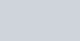• 5
•
•
•
•
•
•
•
5
Shares

# ELECTRIC POTENTIAL DUE TO SYSTEM OF CHARGES

In this article we are going to derive an expression for electric potential due to system of charges. So keep reading…

### DERIVATION FOR THE EXPRESSIONLet’s consider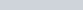are the point charges which are located at the distances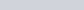respectively from a point P. See figure above. Now electric potential due to charge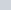is-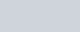Similarly, electric potential due to others charges.By using superposition principle, we can obtain resultant value of electric potential at point P due to total charge configuration as algebraic sum of all the electric potential due to individuals charges.Now taking common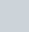from above equation, then we get-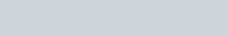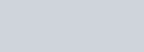The net electric potential due to the multiple charges at the point P is equal to the algebraic sum of all the potential due to individuals charges. Mathematically it is expressed as-